Deutsche VersionSound intensity I and the inverse square law 1/r²

How does the sound intensity decrease with distance from the sound source?
How does the sound intensity level decrease with doubling the distance from the source?
Point sources of gravitational force, electric field, light, or radiation obey the inverse square law.

 Avoid using the psychoacoustical terms loudness perception and volume. This subjective sound-sensation is not clearly measurable without ambiguity. The term "loudness" or "volume" is a problem because it belongs to psycho- acoustics and this personal feeling is not correct definable. Loudness as a psychological correlate of physical strength (amplitude) is also affected by parameters other than sound pressure, including frequency, bandwidth, spectral composition, information content, time structure, and the duration of exposure of the sound signal. The same sound will not create the same loudness perception by all individuals (people).

 As psycho-acoustic parameters to describe the "loudness" there is the "loudness level" with the unit phon and the "loudness" with the unit sone.

Sound pressure p is not sound intensity I.   I ~ p2 is true for progressive plane waves.

Compare also the inverse distance law 1/r, when
using
sound pressure as sound field quantity.

Sound pressure is inversely proportional to the distance of the point of measurement
from the source, so that if we double the distance we halve the sound pressure.

How does the sound decrease with distance?
Damping of sound level with distance

This is an approximation when the venue is a direct sound field or an anechoic room

 In the real world, the inverse square law (squared distance law) I ~ 1/r2 is always an idealization because it assumes exactly equal sound intensity or acoustic intensity I as sound energy propagation in all directions. If there are reflective surfaces in the sound field, then reflected sounds will add to the directed sound and we will get more sound intensity at a field location than the inverse square law predicts. If there are barriers between the source and the point of measurement, we may get less than the square law predicts. Nevertheless, the inverse square law is the logical first estimate of the sound intensity we would get at a distant point in a reasonably open area. The reference sound intensity level SIL = 0 dB is the acoustic intensity of I0 = 1 pW/m2 = 1 × 10−12 W/m2. Note: Since the sound intensity level (energy quantity) is difficult to measure, it is common to use sound pressure level (field quantity) measured in decibels instead. Doubling the sound pressure raises the sound pressure level by 6 dB. Sound pressure in Pa is really not sound intensity in W/m².

 If we measure at a distance  r 1   = m = ft  a sound intensity level (SIL1)  LI1 = dB,  and then at distance  r2   = m = ft  the inverse square law 1/r2 predicts   a sound intensity level (SIL2) of  LI2 = dB in the free field.
 You can explore numerically to confirm the 1/r2 law that doubling the distance drops the sound intensity I to a quarter (0.25) by a sound intensity level of about (−)6 dB and that 10 times the distance drops the sound intensity I to a hundredth (0.01), that is a level drop by 20 dB.

 There is no noise decrease or sound drop per meter. We get a sound level drop of 6 dB per doubling of distance. Sound power or sound power level has nothing to do with the distance from the sound source.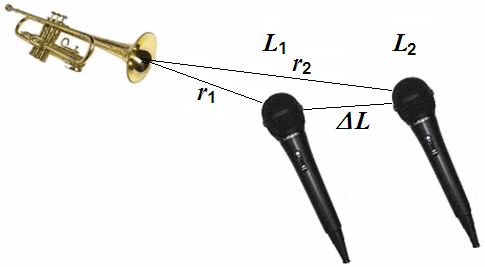Note: The radiated sound power (sound intensity) is the cause and the sound pressure is the effect. where the sound engineer is particularly interested in the effect. The effect of temperature and sound pressure: Sound pressure and Sound power – Effect and Cause.

 Acousticians and sound protectors ("noise fighters") need the sound intensity (acoustic intensity) – but sound engineers and sound designers ("ear people") don't need that sound energy quantity.   Who is involved in audio engineering, should rather take care of the sound field quantity, that is the sound pressure or the sound pressure level (SPL) as an effect at the eardrums of our hearing and on the diaphragms of the microphones, and the corresponding audio voltage and its voltage level.

Sound pressure and Sound power − Effect and Cause

Sound intensity level and Sound intensity

 Enter a value in the left or right box. The calculator works in both directions of the ↔ sign.

 Sound intensity level LI  dB-SIL ↔ Sound intensity I   W/m2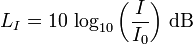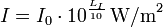Standard reference sound intensity I0 = 1 pW/m2 = 10−12 W/m2 ≡ 0 dB

Inverse square law 1/r2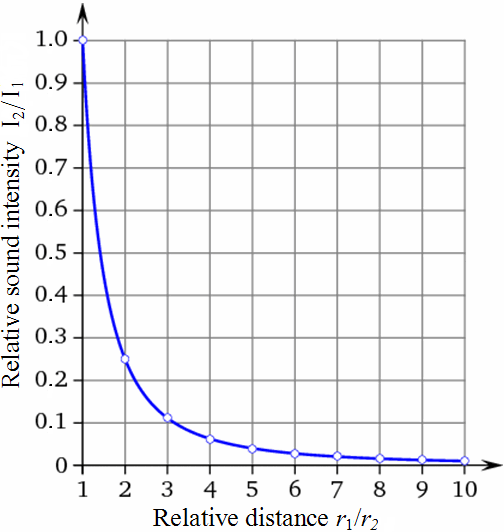Law for sound energy quantities The graphs shown are normalized Distance ratio Sound Intensity I1/r2 1 1/1² = 1/1 = 1.0000 2 1/2² = 1/4 = 0.2500 3 1/3² = 1/9 = 0.1111 4 1/4² = 1/16 = 0.0625 5 1/5² = 1/25 = 0.0400 6 1/6² = 1/36 = 0.0278 7 1/7² = 1/49 = 0.0204 8 1/8² = 1/ 64 = 0.0156 9 1/9² = 1/81 = 0.0123 10 1/10² =1/100 = 0.0100

Calculating sound intensity with the inverse square law

 Relationship of sound intensity I, sound pressure p, and the square law: (r is the distance from the sound source)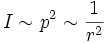This obviously meansFormulas for distance attenuation − Sound intensity calculation

 The value of the sound intensity increases inversely squared with increasing distance from the sound source, that means with 1/r2: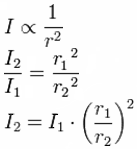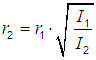Where: I1 = sound intensity 1 at closer distance r1 from the sound source I2 = sound intensity 2 at more far distance r2 from the sound source r1 = closer distance r1 from the sound source r2 = more far distance r2 from the sound source

 Note! Since the sound intensity level is difficult to measure, it is common to use sound pressure level measured in decibels instead. Doubling the sound pressure raises the sound pressure level (SPL) by 6 dB. Doubling the sound intensity raises the sound intensity level by 3 dB.

 The sound pressure level Lp to plot against the distance of the sound source r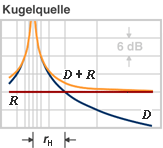D: direct field of the spherical source R: reflected field (diffuse field) rH: critical distance

 If we double the distance, the value for the sound pressure falls to a half (50%) of its initial value. If we double the distance, the value for the sound intensity falls to a quarter (25%) of its initial value. This corresponds to a decrease in level by (−)6 dB. For the level change in dB we get: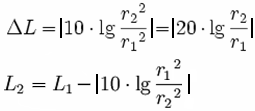A doubling of distance from the sound source in the direct field will reduce the "sound level" by (−)6 dB, no matter whether that are sound intensity levels or sound pressure levels! This will reduce the sound intensity I (energy quantity) to 1/22 = 1/4 (25 %) and the sound pressure p (field quantity) to 1/2 (50 %) of the the initial value. The inverse square law 1/r2 shows the distance performance of energy quantities and the inverse distance law 1/r shows the distance performance of field quantities. Energy quantities are proportional to squared field quantities  –  e.g. I ~ p2.

 How is the sound level dependent from the distance to the sound source?  The sound pressure level shows in the free field situation a reduction of 6 dB per  doubling of distance; that means the sound pressure drops to a half and not a quarter.  It is the sound intensity, that drops to a quarter of the initial value.

 Sound Energy Quantities Sound intensity, sound energy density, sound energy, acoustic  power. (electrical power). Inverse Square Law 1/r² Sound Field Quantities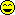Sound pressure, sound or particle velocity, particle displacement or displacement amplitude, (voltage, current, electric resistance). Inverse Distance Law 1/r

Frequently used false statements in the context of
sound values and the distance of the sound source

 Correct version Wrong expression Sound intensity (energy) falls inversely proportional to the square of the distance 1/r2 from the sound source. That is the inverse square law 1/r2. Sound intensity (energy) falls inversely proportional to the distance 1/r from the sound source.                                                    wrong Sound intensity level decreases by (−)6 dB for doubling of the distance from the source to 1/4 (25 %) of the sound intensity initial value. Sound intensity level decreases inversely as the square of the distance increases for doubling of distance from the source by (−)3 dB.         wrong Sound pressure (amplitude) falls inversely proportional to the distance 1/r from the sound source. That is the 1/r law or the inverse distance law. Sound pressure (amplitude) falls inversely proportional to the square of the distance 1/r2 from the sound source.                          Really wrong Sound pressure level decreases by (−)6 dB for doubling of the distance from the source to 1/2 (50 %) of the sound pressure initial value. Sound pressure level decreases inversely as the the distance increases for doubling of distance from the source by (−)3 dB.                             wrong

Sound pressure is not intensity

 Neither the sound power nor the sound power level decreases in doubling the distance. Why is this so? The sound power level quantifies the totally radiated sound energy from an object. Different to the sound pressure the sound power is independent of the distance to the sound source, the surrounding area and other influences.

How many decibels (dB) level change is twice (double, half) or three times as loud?

 Differentiate: Sound pressure p is a "sound field quantity" and sound intensity I is a "sound energy quantity". In teachings these terms are not often separated sharply enough and sometimes are even set equal. But I ~ p2.

Changing of sound power with distance is nonsense

 Question: How does the sound power decrease with distance"? Answer: "April fool - The sound power does not decrease (drop) with distance from the sound source."   Levels of sound pressure and levels of sound intensity decrease equally with the distance from the sound source. Sound power or sound power level has nothing (!) to do with the distance from the sound source. Thinking helps: A 100 watt light bulb has in 1 m and in 10 m distance really always the same 100 watts, which is emitted from the lamp all the time. Watts don't change with distance.   A frequent question: "Does the sound power depend on distance?" The clear answer is: "No, not really."   We consider sound fields in air which are described by the scalar quantity p (sound pressure) and the vector quantity v (sound velocity) as a sound field quantity.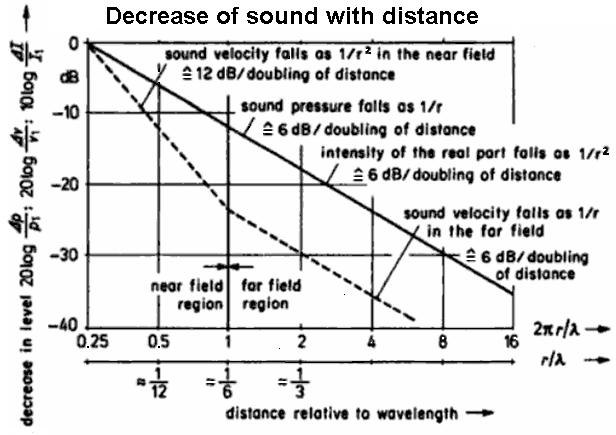Pressure, velocity, and intensity of the sound field near to and distant from a spherical radiator of the zeroth order

 Sound engineers and sound designers (ear people) are mainly interested in sound field quantities and consider more the sound pressure drop at distance doubling (Schalldruckabfall - Entfernungsverdopplung). Acousticians and sound protectors (noise fighters) are mainly interested in sound energy quantities and consider here the sound intensity drop at distance doubling. They all view together the same line!Isn't that beautiful? Nevertheless, the drop in sound intensity goes with 1/r2 and the decrease of sound pressure is 1/r. This should be understood quite well. I ~ p2. Intensity is proportional to the square of the amplitude of the sound pressure.

 Our hearing (eardrum) is directly sensitive to the sound pressure. From the historical perspective, the level differences for stereo listening were called "intensity" differences. However, sound intensity is a specifically defined quantity that can not be picked up by a microphone, nor would it be useful for a sound recording. So call the "intensity" stereophony better level difference stereophony.   If we have to work as a sound technician to check the sound quality by ear, then think of the sound waves, which move the eardrums using the sound pressure as a sound field quantity. There is also the advice: Try to avoid the use of sound power and sound intensity as sound energy quantities.

 In audio, electronics and acoustics use only the word "damping" and not the wrong word "dampening".